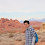## Thursday, June 16, 2016

### Got Data? Adventures in Virtual Crystal Ball Creation

There's two ways to look at this recent Programming Praxis exercise: implementing a beginner level statistics function or creating a magical crystal ball that can predict past, present and future! I chose to approach this problem with the mindset of the latter. Let's make some magic!

The algorithm we're tackling is linear regression. I managed to skip statistics in college (what a shame!), so I don't recall ever being formally taught this technique. Very roughly, if you have the right set of data, you can plot a line through it. You can then use this line to predict values not in the data set.

The exercise gave us this tiny, manufactured, data set:

```x    y
60   3.1
61   3.6
62   3.8
63   4.0
65   4.1
```

With linear regression you can answer questions like: what will be the associated value for say, 64, 66 or 1024 be? Here's my implementation in action:A few words about the screenshot above. You'll notice that I'm converting my data from a simple list to a generator. A generator in this case is a function that will return a single element in the data set, and returns '() when all the data has been exhausted. I chose to use a generator over a simple list because I wanted to allow this solution to scale to large data sets.

Below you'll see a data set that's stored in a file and leverages a file based generator to access its contents. So far, I haven't throw a large data set at this program, but I believe it should scale without issue.

The call to make-crystal-ball performs the linear-regression and returns back a function that when provided x returns a guess prediction for y. What? I'm trying to have a bit of fun here.

Looking around on web I found this example that uses a small, but real data set. In this case, it compares High School and College GPA. Using linear-regression we're able to predict how a High School student with a 2.7, 3.0 or 3.5 GPA is going to do in college. Here's the code:

```;;  http://onlinestatbook.com/2/regression/intro.html
;;  http://onlinestatbook.com/2/case_studies/sat.html
(define (gpa-sat-test)
(define data (file->generator "gpa-sat-data.scm"
(lambda (high-gpa math-sat verb-sat comp-gpa univ-gpa)
(list high-gpa univ-gpa))))
(let ((ball (make-crystal-ball data)))
(show "2.7 => " (ball 2.7))
(show "3.0 => " (ball 3))
(show "3.5 => " (ball 3.5))))
```

And the answer is: 2.91, 3.12 and 3.45 respectively. So yeah, that's good news if you were dragging in High School, you're GPA should climb a bit. But High School overachievers should beware, your GPA is most likely to dip. D'oh.

Below is my implementation of this solution. You can also find it on github. As usual I find myself preaching the benefits of Scheme. The code below was written on my Galaxy Note 5 using Termux, emacs and Tinyscheme. With relative ease I was able to implement a generator framework that works for both lists and data files. I'm also able to leverage the Scheme reader so that the data file format is trivial to operate on. Finally, I wrote a generic sigma function that walks through my data set once, but performs all the various summations I'll need to calculate the necessary values. In other words, I feel like I've got an elegant solution using little more than lists, lambda functions and sexprs. It's beautiful and should be memory efficient.

Here's the code:

```;; https://programmingpraxis.com/2016/06/10/linear-regression/

(define (show . args)
(for-each (lambda (arg)
(display arg)
(display " "))
args)
(newline))

(define (as-list x)
(if (list? x) x (list x)))

(define (g list index)
(list-ref list index))

(define (make-list n seed)
(if (= n 0) '()
(cons seed (make-list (- n 1) seed))))

(define (list->generator lst)
(let ((remaining lst))
(lambda ()
(cond ((null? remaining) '())
(else
(let ((x (car remaining)))
(set! remaining (cdr remaining))
x))))))

(define (file->generator path scrubber)
(let ((port (open-input-file path)))
(lambda ()
(if (eof-object? next) '() (apply scrubber next))))))

(define (sigma generator . fns)
(define (update fns sums data)
(let loop ((fns fns)
(sums sums)
(results '()))
(cond ((null? fns) (reverse results))
(else
(let ((fn (car fns))
(a  (car sums)))
(loop (cdr fns)
(cdr sums)
(cons (+ a (apply fn (as-list data)))
results)))))))

(let loop ((data (generator))
(sums (make-list (length fns) 0)))
(if (null? data) sums
(loop (generator)
(update fns sums data)))))

;; Magic happens here:
;; m = (n × Σxy − Σx × Σy) ÷ (n × Σx2 − (Σx)2)
;; b = (Σy − m × Σx) ÷ n
(define (linear-regression data)
(let ((sums (sigma data
(lambda (x y) (* x y))
(lambda (x y) x)
(lambda (x y) y)
(lambda (x y) (* x x))
(lambda (x y) 1))))
(let* ((Sxy (g sums 0))
(Sx  (g sums 1))
(Sy  (g sums 2))
(Sxx (g sums 3))
(n   (g sums 4)))
(let* ((m (/ (- (* n Sxy) (* Sx Sy))
(- (* n Sxx) (* Sx Sx))))
(b (/ (- Sy (* m Sx)) n)))
(cons m b)))))

(define (make-crystal-ball data)
(let* ((lr (linear-regression data))
(m  (car lr))
(b  (cdr lr)))
(lambda (x)
(+ (* m x) b))))

;; Playtime
(define (test)
(define data (list->generator '((60   3.1)
(61   3.6)
(62   3.8)
(63   4.0)
(65   4.1))))
(let ((ball (make-crystal-ball data)))
(show (ball 64))
(show (ball 66))
(show (ball 1024))))

;; From:
;;  http://onlinestatbook.com/2/regression/intro.html
;;  http://onlinestatbook.com/2/case_studies/sat.html
(define (gpa-sat-test)
(define data (file->generator "gpa-sat-data.scm"
(lambda (high-gpa math-sat verb-sat comp-gpa univ-gpa)
(list high-gpa univ-gpa))))
(let ((ball (make-crystal-ball data)))
(show "2.7 => " (ball 2.7))
(show "3.0 => " (ball 3))
(show "3.5 => " (ball 3.5))))

(gpa-sat-test)

```

1.2.